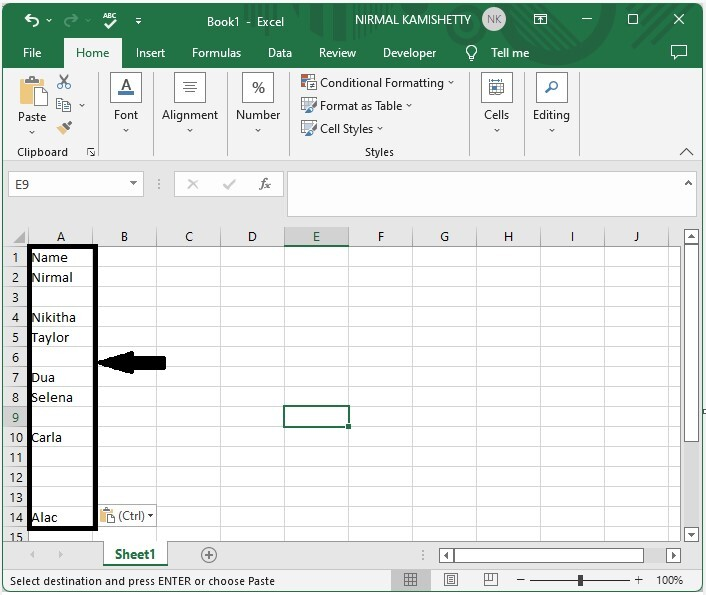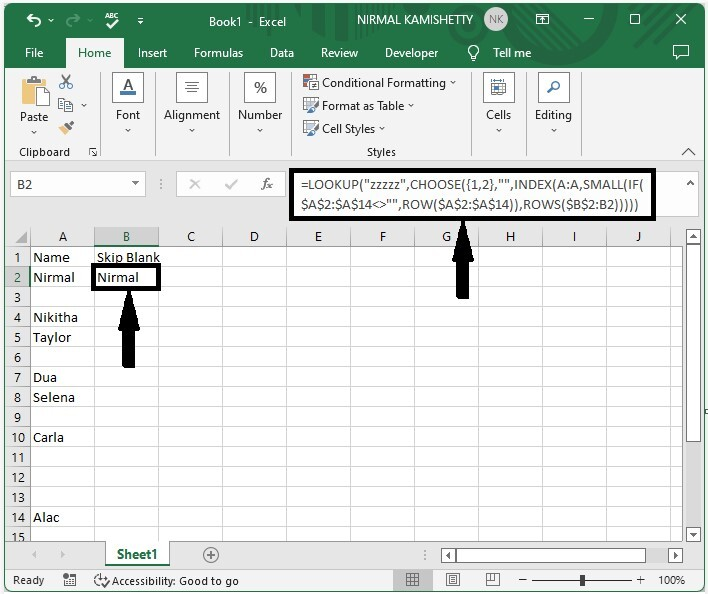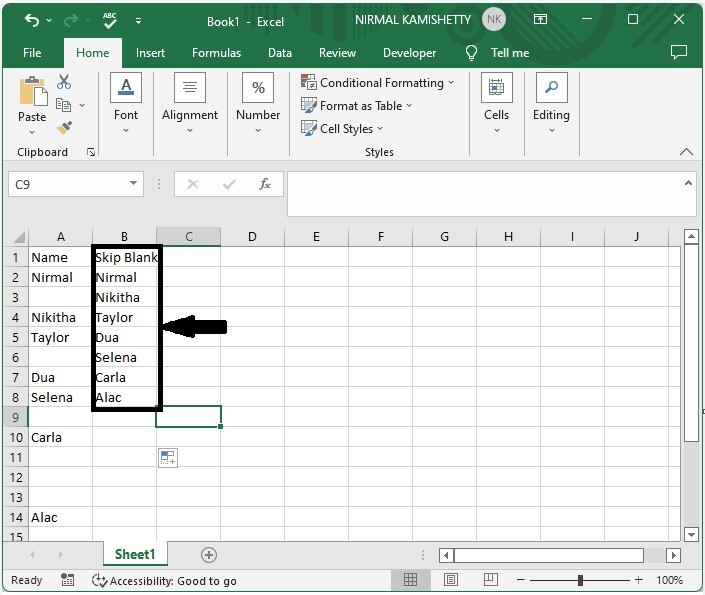# How to Copy and Paste Skip Blank Cells in Excel?

Generally, in Excel, a list may or may not contain empty cells, and when we want to copy the values, the blank cells will also be copied by default. If we need to copy only the cells with data in them by skipping the blank cells, we need to use a special process. If we try to do this manually, it can be time-consuming.

Read this tutorial to learn how you can copy and paste in Excel without skipping blank cells. We can complete this task in a faster and more efficient way by using the array formulas.

## How to Copy and Paste Skip Blank Cells in Excel

Here, we will first use the array formula to get the first value, then fill the whole list using the auto-fill handler. Let's go over a simple procedure for using formulas to copy and paste blank cells in Excel. The formula we're using is an array formula with the LOOKUP function. LOOKUP is one of the reference functions. It is used when you need to look in a single row or column and find a value from the same position in a second row or column.

### Step 1

Consider an Excel sheet with data in the form of a list and empty cells between the elements of the list, as shown in the image below.Now, in our case, cell B2, enter the formula as follows.

=LOOKUP("zzzzz",CHOOSE({1,2},"",INDEX(A:A,SMALL(IF($A$2:$A$14<>"",ROW($A$2:$A$14)),ROWS($B$2:B2))))) and click CTRL + SHIFT + ENTER to get our first result, which looks similar to the below image. In the formula, A2:A14 is the range of cells you want to copy, and B1 is the address of the current cell.

Empty cell > Formula > CTRL + SHIFT + ENTER### Step 2

Then drag down from the first result till empty cells are printed, and our final result will be similar to the below image.## Conclusion

In this tutorial, we used a simple example to demonstrate how we can copy and paste blank cells to highlight a particular set of data in Excel.